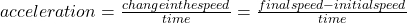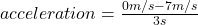## A skateboarder traveling at 7.0 meters per second rolls to a stop at the top of a ramp in 3.0 seconds. What is the skateboarder’s accelerati

Question

A skateboarder traveling at 7.0 meters per second rolls to a stop at the top of a ramp in 3.0 seconds. What is the skateboarder’s acceleration? acceleration=[acceleration]m/s2

in progress 0
7 months 2021-07-13T04:51:53+00:00 1 Answers 12 views 0

## Answers ( )

The skateboarder’s acceleration is -2.33 m/s².

Explanation:

The acceleration of an object is a magnitude that indicates how the speed of the object changes in a unit of time. That is, acceleration is the change in speed in a unit of time.

Acceleration is calculated as the relationship between the variation or change in the speed of a mobile and the time used for said change:In this case:

• final speed= 0 m/s
• initial speed= 7 m/s
• time= 3 s

Replacing:and solving you get:

acceleration= -2.33 m/s²

The skateboarder’s acceleration is -2.33 m/s².